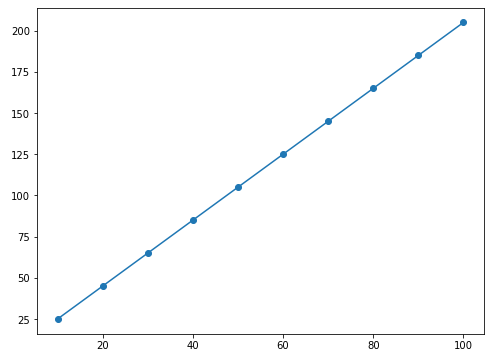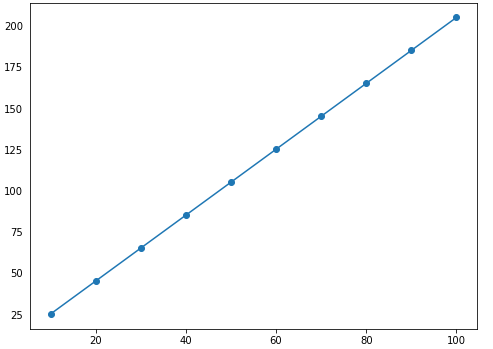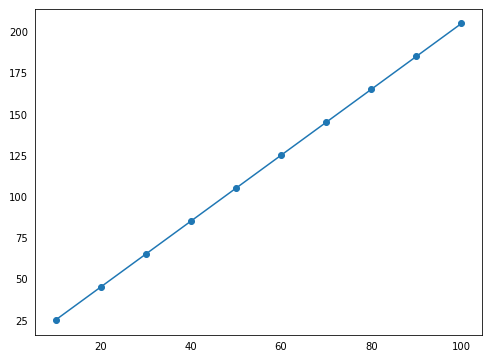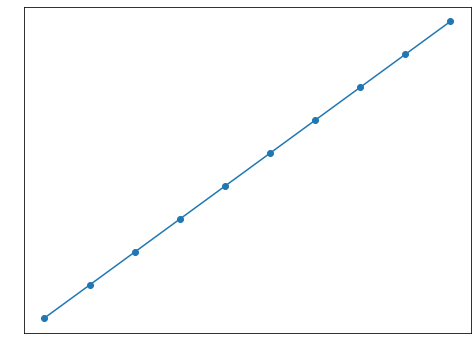Open in App
Not now

# How to Remove Ticks from Matplotlib Plots?

• Difficulty Level : Basic
• Last Updated : 24 Feb, 2021

Matplotlib is a Python library that offers us various functions using which we can plot our data and visualize it graphically. But often plotting a graph using the Matplotlib library, we get ticks in our plot which are marked by default on both sides of the plot on the x and y-axis. There can be cases when we don’t want to show these ticks in our plot. Matplotlib.pyplot library offers us a tick_params() method using which we can remove these ticks manually.

The tick_params() function accepts some attributes that take Boolean values which can be used to remove ticks and labels on the plot.  By default, the values in this attribute are set to True,

Let’s understand this using an example:

## Python

 `# importing matplotlib library``import` `matplotlib.pyplot as plt`` ` `# making points to be plotted on x-axis and y-axis``X_axis ``=` `[i ``for` `i ``in` `range` `(``10``, ``110``, ``10``)]``Y_axis ``=` `[``2``*``j``+``5` `for` `j ``in` `range` `(``10``, ``110``, ``10``)]`` ` `# printing points to be plotted on the x and y-axis``print``(``"Points on x-axis are: "``,X_axis)``print``(``"Points on y-axis are: "``,Y_axis)`` ` `# Creating a default plot``plt.figure(figsize``=``(``8``,``6``))``plt.plot(X_axis,Y_axis)``plt.scatter(X_axis,Y_axis)``plt.show()`

Output:

Points on x-axis are:  [10, 20, 30, 40, 50, 60, 70, 80, 90, 100]

Points on y-axis are:  [25, 45, 65, 85, 105, 125, 145, 165, 185, 205]default plot made using matplotlib including ticks

By viewing the above image, we can observe by default Matplotlib marks ticks on the x and y-axis.

Case 1.1: When we want to remove ticks on a single axis (here y-axis):

To remove the ticks on the y-axis, tick_params() method has an attribute named left and we can set its value to False and pass it as a parameter inside the tick_params() function. It removes the tick on the y-axis.

## Python

 `# importing matplotlib library``import` `matplotlib.pyplot as plt`` ` `# making points to be plotted on x-axis and y-axis``X_axis ``=` `[i ``for` `i ``in` `range` `(``10``, ``110``, ``10``)]``Y_axis ``=` `[``2``*``j``+``5` `for` `j ``in` `range` `(``10``, ``110``, ``10``)]`` ` `# printing points to be plotted on the x and y-axis``print``(``"Points on x-axis are: "``, X_axis)``print``(``"Points on y-axis are: "``, Y_axis)`` ` `plt.figure(figsize ``=` `( ``8``, ``6``))``plt.tick_params(left ``=` `False``)``plt.plot(X_axis, Y_axis)``plt.scatter(X_axis, Y_axis)``plt.show()`

Output:plot made after using tick_params() method to remove ticks on y-axis

Case 1.2: When we want to remove ticks on a single axis (here x-axis):

To remove the ticks on the x-axis, tick_params() method accepts an attribute named bottom, and we can set its value to False and pass it as a parameter inside the tick_params() function. It removes the tick on the x-axis.

## Python

 `# importing matplotlib library``import` `matplotlib.pyplot as plt`` ` `# making points to be plotted on x-axis and y-axis``X_axis ``=` `[i ``for` `i ``in` `range` `(``10``, ``110``, ``10``)]``Y_axis ``=` `[``2``*``j``+``5` `for` `j ``in` `range` `(``10``, ``110``, ``10``)]`` ` `# printing points to be plotted on the x and y-axis``print``(``"Points on x-axis are: "``, X_axis)``print``(``"Points on y-axis are: "``, Y_axis)`` ` `plt.figure(figsize ``=` `(``8``, ``6``))``plt.tick_params(bottom ``=` `False``)``plt.plot(X_axis, Y_axis)``plt.scatter(X_axis, Y_axis)``plt.show()`

Output:plot made after using tick_params() method to remove ticks on x-axis

Case 2: Removing these ticks on both the axes using tick_params() method:

To remove the ticks on both the x-axis and y-axis simultaneously, we can pass both left and right attributes simultaneously setting its value to False and pass it as a parameter inside the tick_params() function. It removes the ticks on both x-axis and the y-axis simultaneously.

## Python

 `# importing matplotlib library``import` `matplotlib.pyplot as plt`` ` `# making points to be plotted on x-axis and y-axis``X_axis ``=` `[i ``for` `i ``in` `range` `(``10``, ``110``, ``10``)]``Y_axis ``=` `[``2``*``j``+``5` `for` `j ``in` `range` `(``10``, ``110``, ``10``)]`` ` `# printing points to be plotted on the x and y-axis``print``(``"Points on x-axis are: "``, X_axis)``print``(``"Points on y-axis are: "``, Y_axis)`` ` `plt.figure(figsize ``=` `(``8``,``6``))``plt.tick_params(left ``=` `False``, bottom ``=` `False``)``plt.plot(X_axis, Y_axis)``plt.scatter(X_axis, Y_axis)``plt.show()`

Output:plot made after using tick_params() method to remove ticks on both x and y-axis

Case 3: When we want to remove both ticks and labels from both the axes

To remove both ticks and labels from both the axes simultaneously, apart from setting both left and right attributes to False, we will also use two additional attributes for labels which are- labelleft and labelbottom and we will set its value to False and pass it inside the tick_params() function. It will remove labels from both axes also.

## Python3

 `# importing matplotlib library``import` `matplotlib.pyplot as plt`` ` `# making points to be plotted on x-axis and y-axis``X_axis ``=` `[i ``for` `i ``in` `range` `(``10``, ``110``, ``10``)]``Y_axis ``=` `[``2``*``j``+``5` `for` `j ``in` `range` `(``10``, ``110``, ``10``)]`` ` `# printing points to be plotted on the x and y-axis``print``(``"Points on x-axis are: "``, X_axis)``print``(``"Points on y-axis are: "``, Y_axis)`` ` `plt.figure(figsize ``=` `(``8``,``6``))``plt.tick_params(left ``=` `False``, right ``=` `False` `, labelleft ``=` `False` `,``                ``labelbottom ``=` `False``, bottom ``=` `False``)`` ` `plt.plot(X_axis, Y_axis)``plt.scatter(X_axis, Y_axis)``plt.show()`

Output:plot made after using tick_params() method to remove ticks  and labels on both x and y-axis

My Personal Notes arrow_drop_up